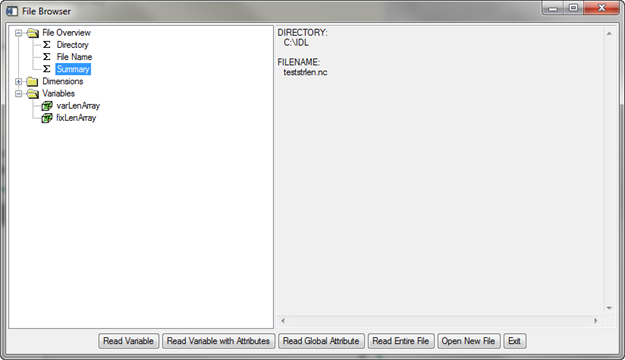Writing Fixed Length Strings Into netCDF FilesQUESTION: Does anyone know if it is possible to write an array of strings to a variable in a netCDF-4 file as some fixed-length string that has a length greater than one? Let me show what I mean. I would like fixed length strings of length 10.

Let's define a string array.

array = ['cat', 'coyote', 'elephant']

Here is one method for writing the string array.

id = NCDF_CREATE('myfile.nc', /CLOBBER, /NETCDF4_FORMAT)
dim1 = NCDF_DIMDEF(id,'dim1', 3)
len_dim = NCDF_DIMDEF(id, 'string length', 10)
vid = NCDF_VARDEF(id, 'Names', [len_dim, dim1], /CHAR)
NCDF_CONTROL, id, /ENDEF
NCDF_VARPUT, id, vid, array
NCDF_CLOSE, id

This results in a 2D array of CHAR, with dimension (10,3).

This is a second method for writing the string array.

id = NCDF_CREATE('myfile2.nc', /CLOBBER, /NETCDF4_FORMAT)
dim1 = NCDF_DIMDEF(id,'dim1', 3)
vid = NCDF_VARDEF(id, 'Names', [dim1], /STRING)
NCDF_CONTROL, id, /ENDEF
NCDF_VARPUT, id, vid, array
NCDF_CLOSE, id

This results in a vector of variable-length strings, with three elements. What I really want is vector of fixed-length strings, with three elements, each element having a string length of 10. Can you show me how to do this?ANSWER: I'm afraid I don't often work with netCDF files, and when I do I find the documentation totally confusing. At one point in my life, I worked with netCDF files all the time, so to avoid constant confusion, I wrote my own netCDF routines to work with netCDF files. So, I can't actually show you how to do this using the built-in IDL commands, but I can show you how to do it using the nCDF_File object I created to work with these kinds of files. You can see how the object is used in this program of netCDF examples.

I would put fixed length strings into a netCDF file by making the strings a fixed length in IDL before I wrote them to the file. So, first, I would do this to create an array of fixed length strings of length 10.

array = ['cat', 'coyote', 'elephant']
fixedLenArray = String(array, Format='(A10)')

Let's write both of these arrays into the file, so we can see the difference. First, we open the file and set up the dimensions that describe the data files.

fileObj = Obj_New('ncdf_file', 'teststrlen.nc', /Clobber, /Create, /NETCDF4_FORMAT)
fileObj -> WriteDim, 'xdim', , OBJECT=xdimObj
fileObj -> WriteDim, 'ydim', , OBJECT=ydimObj
dimNames = [xdimObj->GetName(), ydimObj->GetName()]

Next, we define the variable definitions.

fileObj -> WriteVarDef, 'varLenArray', dimNames, DATATYPE='STRING', OBJECT=varObj
fileObj -> WriteVarDef, 'fixLenArray', dimNames, DATATYPE='STRING', OBJECT=fixObj

Next, we write the data to the file and make sure there are no buffering issues by syncing the file.

fileObj -> WriteVarData, varObj, array
fileObj -> WriteVarData, fixObj, fixedLenArray
fileObj -> Sync

Finally, since we are done with the file, we destroy the object.

Obj_Destroy, fileObj

Let's view the file with the nCDF_Browser program from the Coyote Library.

nCDF_Browser, 'teststrlen.nc'

You see the results in the figure below. You can read the two variables out of the file using the buttons of the browser, or you can do this programmatically, as I will demonstrate below the figure.The two string arrays shown in the nCDF_Browser.

To read the data out of the file programmatically, type these commands.

fileObj = Obj_New('ncdf_file', 'teststrlen.nc')
v1 = fileObj -> GetVarData('varLenArray')
v2 = fileObj -> GetVarData('fixLenArray')
Obj_Destroy, fileObj

To see the difference between these two variables, type these commands.

IDL> Help, v1, v2
V1              STRING    = Array
V2              STRING    = Array
IDL> FOR j=0,2 DO Print, 'String Length: ', StrTrim(StrLen(v1[j]),2), ' ', v1[j]
String Length: 3 cat
String Length: 6 coyote
String Length: 8 elephant
IDL> FOR j=0,2 DO Print, 'String Length: ', StrTrim(StrLen(v2[j]),2), ' ', v2[j]
String Length: 10        cat
String Length: 10     coyote
String Length: 10   elephant

You can see clearly that the variable v2 contains a vector of fixed length strings.